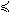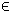## 16SDP Problem

The linear semi-definite programming problem with linear matrix inequalities (sdp) is defined as

 min x
f(x) = cTx

s/t xL x xU
bL Ax bU

Q0i +
 Σ k=1
n Qkixk0,     i=1,…,m.
(18)
where c, x, xL, xURn, ARml × n, bL,bURml and Qki are symmetric matrices of similar dimensions in each constraint i. If there are several LMI constraints, each may have it's own dimension.

The following file defines and solves a problem in TOMLAB.

File: tomlab/quickguide/sdpQG.m

Open the file for viewing, and execute sdpQG in Matlab.

This problem appears to be infeasible.
```% sdpQG is a small example problem for defining and solving
% semi definite programming problems with linear matrix
% inequalities using the TOMLAB format.

Name = 'sdp.ps example 2';

% Objective function
c = [1 2 3]';

% Two linear constraints
A =   [ 0 0 1 ; 5 6 0 ];
b_L = [-Inf; -Inf];
b_U = [ 3  ; -3 ];

x_L = -1000*ones(3,1);
x_U =  1000*ones(3,1);

% Two linear matrix inequality constraints. It is OK to give only
% the upper triangular part.
SDP = [];
% First constraint
SDP(1).Q{1} = [2 -1 0 ; 0 2 0 ; 0 0 2];
SDP(1).Q{2} = [2 0 -1 ; 0 2 0 ; 0 0 2];
SDP(1).Qidx = [1; 3];

% Second constraint
SDP(2).Q{1} = diag( [0  1] );
SDP(2).Q{2} = diag( [1 -1] );
SDP(2).Q{3} = diag( [3 -3] );
SDP(2).Qidx = [0; 1; 2];

x_0 = [];

Prob = sdpAssign(c, SDP, A, b_L, b_U, x_L, x_U, x_0, Name);

Result = tomRun('pensdp', Prob, 1);```